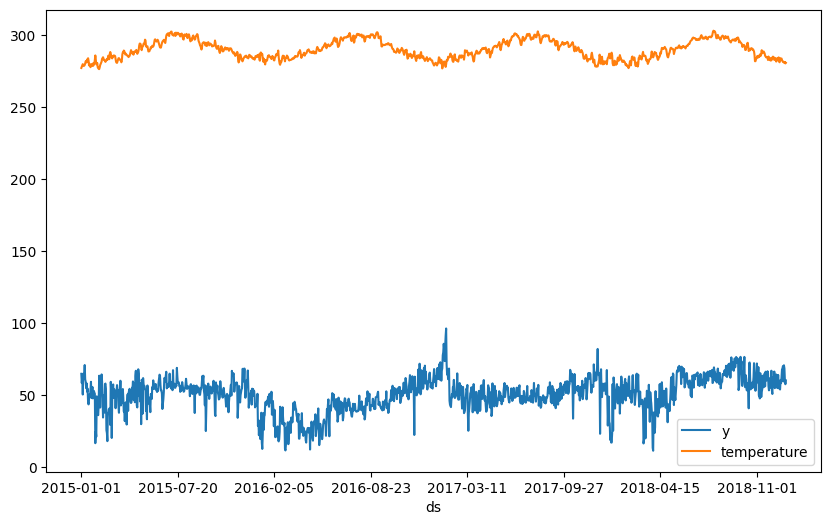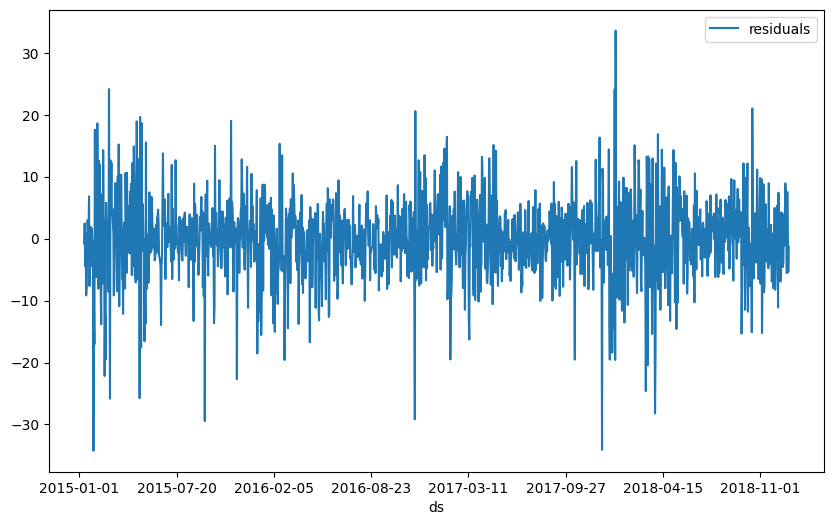# Tutorial 6: Future regressors#

To model future regressors, both past and future values of these regressors have to be known. So in contrast to the lagged regressors in the previous tutorial, future regressors also have a forecasted value for the future in addition to the historic values.

:

import pandas as pd

# Load the dataset for tutorial 4 with the extra temperature column

:

ds y temperature
0 2015-01-01 64.92 277.00
1 2015-01-02 58.46 277.95
2 2015-01-03 63.35 278.83
3 2015-01-04 50.54 279.64
4 2015-01-05 64.89 279.05
:

fig = df.plot(x="ds", y=["y", "temperature"], figsize=(10, 6)):

from neuralprophet import NeuralProphet, set_log_level

# Disable logging messages unless there is an error
set_log_level("ERROR")

# Model and prediction
m = NeuralProphet(
# Disable trend changepoints
n_changepoints=10,
# Disable seasonality components
yearly_seasonality=True,
weekly_seasonality=True,
daily_seasonality=True,
n_lags=10,
)
m.set_plotting_backend("plotly-static")

# Add the new future regressor

# Continue training the model and making a prediction
metrics = m.fit(df)
forecast = m.predict(df)
m.plot(forecast):

m.plot_components(forecast, components=["future_regressors"]):

m.plot_parameters(components=["future_regressors"]):

metrics

:

MAE RMSE Loss RegLoss epoch
0 36.102451 45.784710 0.222547 0.0 0
1 33.973568 43.057194 0.200457 0.0 1
2 32.275692 41.063568 0.183792 0.0 2
3 29.880583 38.180260 0.161229 0.0 3
4 27.537054 35.115955 0.138702 0.0 4
... ... ... ... ... ...
168 4.842709 6.438596 0.005013 0.0 168
169 4.883170 6.518759 0.005086 0.0 169
170 4.834648 6.401788 0.005007 0.0 170
171 4.839586 6.429725 0.005016 0.0 171
172 4.868096 6.472027 0.005059 0.0 172

173 rows × 5 columns

:

df_residuals = pd.DataFrame({"ds": df["ds"], "residuals": df["y"] - forecast["yhat1"]})
fig = df_residuals.plot(x="ds", y="residuals", figsize=(10, 6))